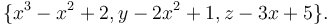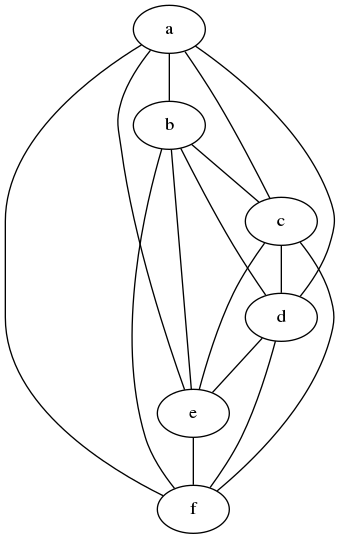(diff) ← Older revision | Latest revision (diff) | Newer revision → (diff)

## Specialized Markup Types

The Advanced Features feature of the Open Risk Manual include:

• Rich Text and specialized content creation facilities. Currently the list of support comprises of the following:
• HTML Markup. To be used sparingly, e.g., when standard mediawiki markup does not offer sufficient flexibility
• Code syntax highlighting. To be used when including a code snippet
• Latex Notation for mathematics. To be used for equations or other mathematical notation
• Graph Diagrams. To be used when creating graphs, networks, trees or other such visual depiction of relationships
• Semantic Data. The Open Risk Manual is based on the Semantic Mediawiki platform. The platform implements features of the Semantic Web, an extension of common web standards provides that allows data to be shared and reused across application, enterprise, and community boundaries. NB. This feature is still experimental, see for example: Semantic Documentation Example

### HTML Markup

HTML Markup can be included by enclosing html in a tag as follows:

```1 <html>
2 <ul>
3 <li> One </li>
4 <li> Two </li>
5 </ul>
6 </html>
```

which produces:

• One
• Two

### Code Snippets

Code for in a large variety of programming languages can be incuded using

```def quickSort(arr):
less = []
pivotList = []
more = []
if len(arr) <= 1:
return arr
else:
pass
```

which produces

```1 def quickSort(arr):
2 	less = []
3 	pivotList = []
4 	more = []
5 	if len(arr) <= 1:
6 		return arr
7 	else:
8 		pass
```

## Mathematics

Mathematics can be included using latex notation. For example:

```:[itex]
\{x^3 - x^2+2, y - 2x^2 +1, z - 3x+5\}.
[/itex]
```

Renders as$\{x^3 - x^2+2, y - 2x^2 +1, z - 3x+5\}.$

## Graph Diagrams

Visual illustrations of concepts can be included using any of the GraphViz supported formats. For example the following graph expressed in dot language

```graph {
a -- b;
b -- c;
c -- d;
d -- e;
e -- f;
a -- f;
a -- c;
a -- d;
a -- e;
b -- d;
b -- e;
b -- f;
c -- e;
c -- f;
d -- f;
}
```

will be rendered as